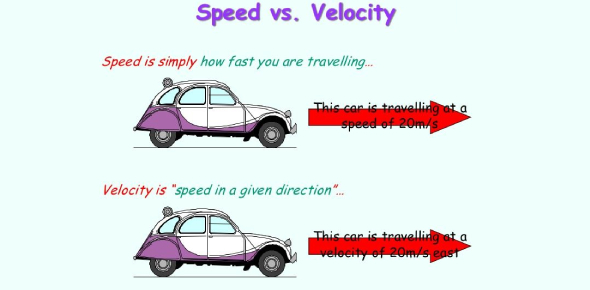# Quiz On Speed And Velocity! Trivia

8 Questions | Total Attempts: 54Settings.

• 1.
Determines how far something tracked in a given amount of time, the SI unit is measured in m/s.
• A.

Speed

• B.

Average velocity

• C.

Vector

• 2.
The measure of the passing of seconds, minutes, hours, years, decades, or centuries; typically measured in seconds for the purpose of experimentation.
• A.

Speed

• B.

Time

• C.

Average

• 3.
Average speed = distance                            -------------                            Time taken
• A.

True

• B.

False

• 4.
Average velocity is
• A.

Maintaining a constant rate of speed.

• B.

Total distance traveled by the total time taken.

• C.

A vector.

• 5.
Constant velocity: Maintaining a constant rate of speed.
• A.

True

• B.

False

• 6.
Speed at any given instant. The SI unit is measured in m/s is...
• A.

Speed

• B.

Time

• C.

Instantaneous speed

• 7.
The measure of both speed and direction is
• 8.
The measurement that includes both size and direction is a
Related TopicsBack to top